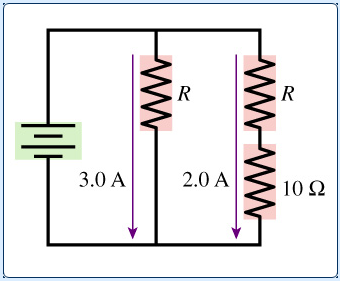# Problem: What is the resistance R in the figure?What is the emf of the battery in the figure?

###### FREE Expert Solution

a)

$\overline{){\mathbf{V}}{\mathbf{=}}{\mathbf{I}}{\mathbf{R}}}$

The Potential difference across the junction is the same.

3.0 A × R = (2.0 A × R) + (2.0 A × 10 Ω)

3R - 2R = 20

99% (29 ratings)###### Problem DetailsWhat is the resistance R in the figure?

What is the emf of the battery in the figure?